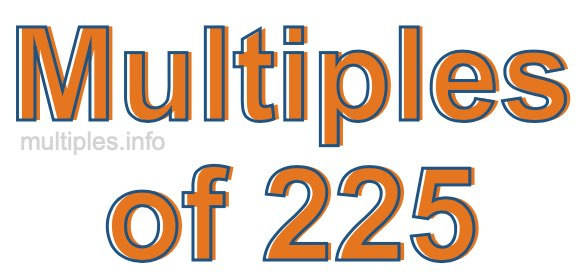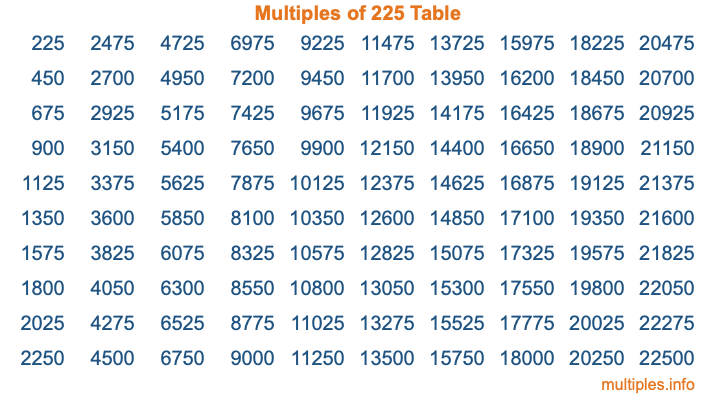Multiples of 225Welcome to the Multiples of 225 page. Here we will first teach you everything you will ever need to know about the multiples of 225, and then give you a study guide summary of everything we taught you to make sure you remember it all. Use this page to look up facts and learn information about the multiples of 225. This page will make you a multiples of two hundred twenty-five expert!

Definition of Multiples of 225
Multiples of 225 are all the numbers that when divided by 225 equal an integer. Each of the multiples of 225 are called a multiple. A multiple of 225 is created by multiplying 225 by an integer.

Therefore, to create a list of multiples of 225, you start with 1 multiplied by 225, then 2 multiplied by 225, then 3 multiplied by 225, and so on for as long as you want. Thus, the list of the first five multiples of 225 is 225, 450, 675, 900, and 1125. To see a larger list of multiples of 225, see the printable image of Multiples of 225 further down on this page. We also have a category where you can choose any nth multiple of 225.

Multiples of 225 Checker
The Multiples of 225 Checker below checks to see if any number of your choice is a multiple of 225. In other words, it checks to see if there is any number (integer) that when multiplied by 225 will equal your number. To do that, we divide your number by 225. If the the quotient is an integer, then your number is a multiple of 225.

Is  a multiple of 225?

Least Common Multiple of 225 and ...
A Least Common Multiple (LCM) is the lowest multiple that two or more numbers have in common. This is also called the smallest common multiple or lowest common multiple and is useful to know when you are adding our subtracting fractions. Enter one or more numbers below (225 is already entered) to find the LCM.

Check out our LCM Calculator if you need more details about the Least Common Multiple or if you need the LCM for different numbers for adding and subtraction fractions.

nth Multiple of 225
As we stated above, 225 is the first multiple of 225, 450 is the second multiple of 225, 675 is the third multiple of 225, and so on. Enter a number below to find the nth multiple of 225.

th multiple of 225

Multiples of 225 vs Factors of 225
225 is a multiple of 225 and a factor of 225, but that is where the similarities end. All postive multiples of 225 are 225 or greater than 225. All positive factors of 225 are 225 or less than 225.

Below is the beginning list of multiples of 225 and the factors of 225 so you can compare:

Multiples of 225: 225, 450, 675, 900, 1125, etc.

Factors of 225: 1, 3, 5, 9, 15, 25, 45, 75, 225

As you can see, the multiples of 225 are all the numbers that you can divide by 225 to get a whole number. The factors of 225, on the other hand, are all the whole numbers that you can multiply by another whole number to get 225.

It's also interesting to note that if a number (x) is a factor of 225, then 225 will also be a multiple of that number (x).

Multiples of 225 vs Divisors of 225
The divisors of 225 are all the integers that 225 can be divided by evenly. Below is a list of the divisors of 225.

Divisors of 225: 1, 3, 5, 9, 15, 25, 45, 75, 225

The interesting thing to note here is that if you take any multiple of 225 and divide it by a divisor of 225, you will see that the quotient is an integer.

Multiples of 225 Table
Below is an image of the first 100 multiples of 225 in a table. The table is in chronological order, column by column. The first column has the first ten multiples of 225, the second column has the next ten multiples of 225, and so on.The Multiples of 225 Table is also referred to as the 225 Times Table or Times Table of 225. You are welcome to print out our table for your studies.

Negative Multiples of 225
Although not often discussed or needed in math, it is worth mentioning that you can make a list of negative multiples of 225 by multiplying 225 by -1, then by -2, then by -3, and so on, to get the following list of negative multiples of 225:

-225, -450, -675, -900, -1125, etc.

Multiples of 225 Summary
Below is a summary of important Multiples of 225 facts that we have discussed on this page. To retain the knowledge on this page, we recommend that you read through the summary and explain to yourself or a study partner why they hold true.

There are an infinite number of multiples of 225.

A multiple of 225 divided by 225 will equal a whole number.

225 divided by a factor of 225 equals a divisor of 225.

The nth multiple of 225 is n times 225.

The largest factor of 225 is equal to the first positive multiple of 225.

225 is a multiple of every factor of 225.

225 is a multiple of 225.

A multiple of 225 divided by a divisor of 225 equals an integer.

225 divided by a divisor of 225 equals a factor of 225.

Any integer times 225 will equal a multiple of 225.

Multiples of a Number
Here you can get the multiples of another number, all with the same attention to detail as we did for multiples of 225 on this page.

Multiples of
Multiples of 226
Did you find our page about multiples of two hundred twenty-five educational? Do you want more knowledge? Check out the multiples of the next number on our list!